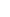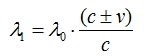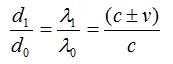14. ALICE EQUATIONSWe have seen that wavelength change in Doppler Shift is as follows:λ0 = Factory setting of wavelength λ1 = Wavelength changeWe have also seen the relationship between distances and signal speeds in this topic:d0 = Distance INCOMING signal covers relative to the receiver c = Speed of INCOMING signal relative to the receiver d1 = Distance OUTGOING signal covers relative to the transmitter  (c+v) = Speed of OUTGOING signal relative to the transmitterTherefore;  is obtained from  and .And we obtain equations ,  and  from . I named these Alice Equations.1) Wavelength change based on distances:2) Calculation methods of v value: